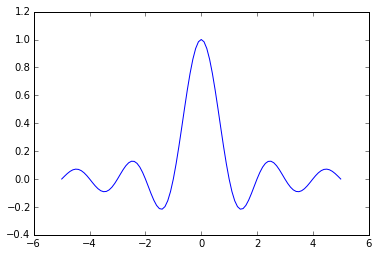# 向量化函数¶

In :
import numpy as np

def sinc(x):
if x == 0.0:
return 1.0
else:
w = np.pi * x
return np.sin(w) / w


In :
sinc(0.0)

Out:
1.0
In :
sinc(3.0)

Out:
3.8981718325193755e-17

In :
x = np.array([1,2,3])
sinc(x)

---------------------------------------------------------------------------
ValueError                                Traceback (most recent call last)
<ipython-input-4-9d4f36f2aa7a> in <module>()
1 x = np.array([1,2,3])
----> 2 sinc(x)

<ipython-input-1-dffe464e3332> in sinc(x)
2
3 def sinc(x):
----> 4     if x == 0.0:
5         return 1.0
6     else:

ValueError: The truth value of an array with more than one element is ambiguous. Use a.any() or a.all()

In :
vsinc = np.vectorize(sinc)
vsinc(x)

Out:
array([  3.89817183e-17,  -3.89817183e-17,   3.89817183e-17])

In :
import matplotlib.pyplot as plt
%matplotlib inline

x = np.linspace(-5,5,101)
plt.plot(x, vsinc(x))

Out:
[<matplotlib.lines.Line2D at 0xa24e4e0>]